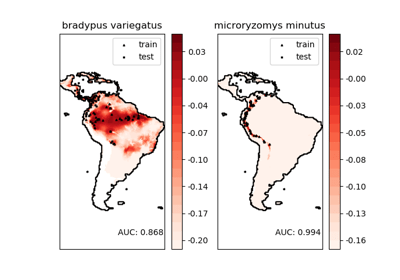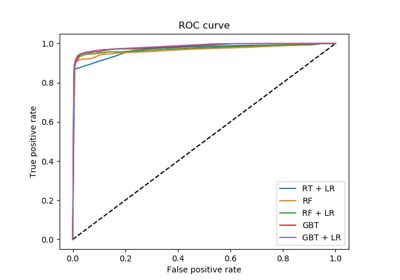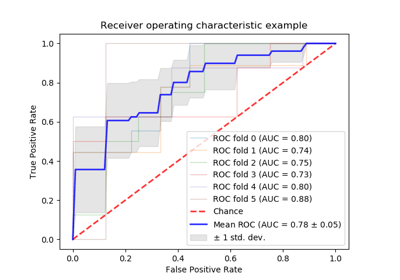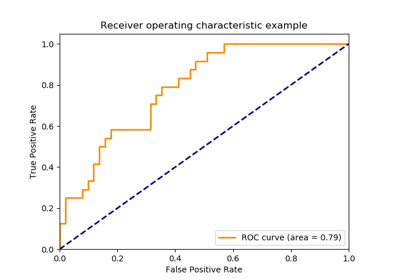/scikit-learn

# sklearn.metrics.roc_curve

`sklearn.metrics.roc_curve(y_true, y_score, pos_label=None, sample_weight=None, drop_intermediate=True)` [source]

Note: this implementation is restricted to the binary classification task.

Read more in the User Guide.

Parameters: `y_true : array, shape = [n_samples]` True binary labels. If labels are not either {-1, 1} or {0, 1}, then pos_label should be explicitly given. `y_score : array, shape = [n_samples]` Target scores, can either be probability estimates of the positive class, confidence values, or non-thresholded measure of decisions (as returned by “decision_function” on some classifiers). `pos_label : int or str, default=None` Label considered as positive and others are considered negative. `sample_weight : array-like of shape = [n_samples], optional` Sample weights. `drop_intermediate : boolean, optional (default=True)` Whether to drop some suboptimal thresholds which would not appear on a plotted ROC curve. This is useful in order to create lighter ROC curves. New in version 0.17: parameter drop_intermediate. `fpr : array, shape = [>2]` Increasing false positive rates such that element i is the false positive rate of predictions with score >= thresholds[i]. `tpr : array, shape = [>2]` Increasing true positive rates such that element i is the true positive rate of predictions with score >= thresholds[i]. `thresholds : array, shape = [n_thresholds]` Decreasing thresholds on the decision function used to compute fpr and tpr. `thresholds` represents no instances being predicted and is arbitrarily set to `max(y_score) + 1`.

`roc_auc_score`
Compute the area under the ROC curve

#### Notes

Since the thresholds are sorted from low to high values, they are reversed upon returning them to ensure they correspond to both `fpr` and `tpr`, which are sorted in reversed order during their calculation.

#### References

  Fawcett T. An introduction to ROC analysis[J]. Pattern Recognition Letters, 2006, 27(8):861-874.

#### Examples

```>>> import numpy as np
>>> from sklearn import metrics
>>> y = np.array([1, 1, 2, 2])
>>> scores = np.array([0.1, 0.4, 0.35, 0.8])
>>> fpr, tpr, thresholds = metrics.roc_curve(y, scores, pos_label=2)
>>> fpr
array([0. , 0. , 0.5, 0.5, 1. ])
>>> tpr
array([0. , 0.5, 0.5, 1. , 1. ])
>>> thresholds
array([1.8 , 0.8 , 0.4 , 0.35, 0.1 ])
```

## Examples using `sklearn.metrics.roc_curve`Species distribution modelingFeature transformations with ensembles of treesReceiver Operating Characteristic (ROC) with cross validation